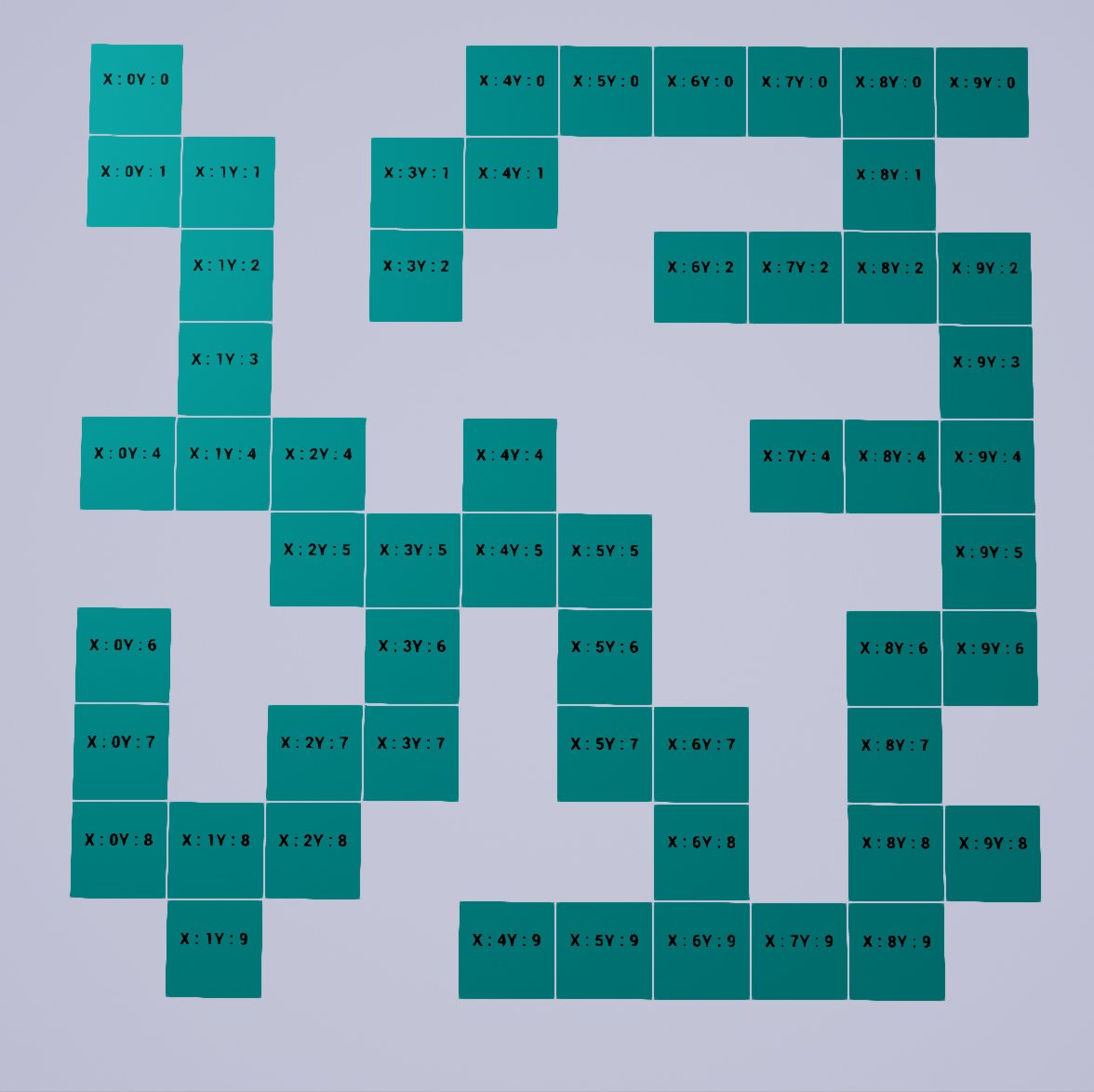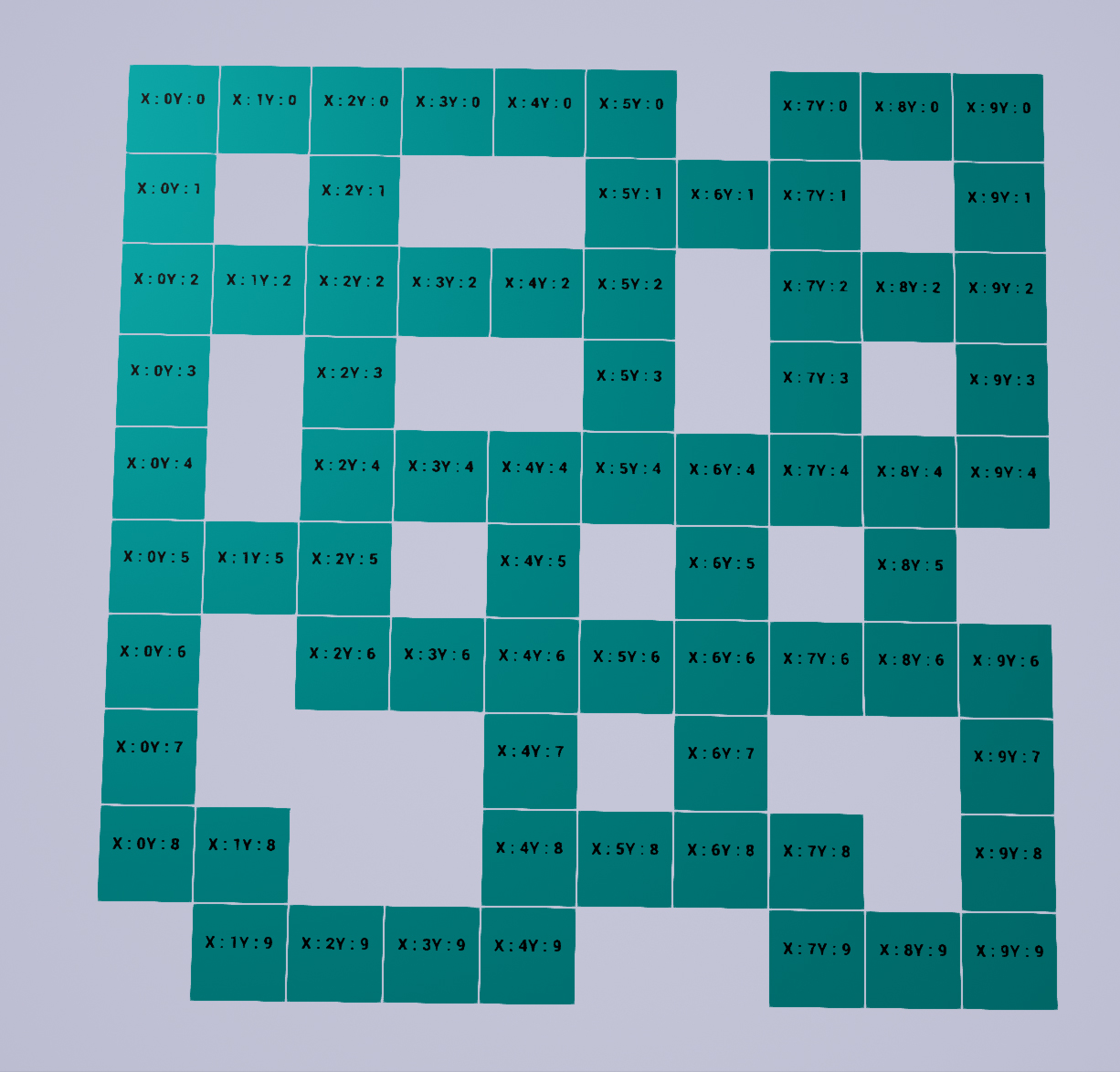# Randomly generated dungeon – Part II

### Part two …

In part one we figured what tiles to place, we got some help trough some visual aid. In this part I thought I might show you how I went about setting up the blueprints.
This is pretty straight forward.

I am making everything in construction script right now.

So I start by making a bunch of integer variables that I know I will need.

• int : GridX (how many tiles in x, default 5)
• int : GridY (how many tiles in Y, default 5)
• int : TileSize (size of tile, default 100)
• vector2d : Mazearray (this is an array that I add all the tiles that I can move to, or create if you will)
• int : CurrentileX and Y (the tile we are standing on)
• int : StartTileX and Y (starting coordinates, default 0,0)

First thing you want to do is to add the start tile. So we add that to the array.  Voila..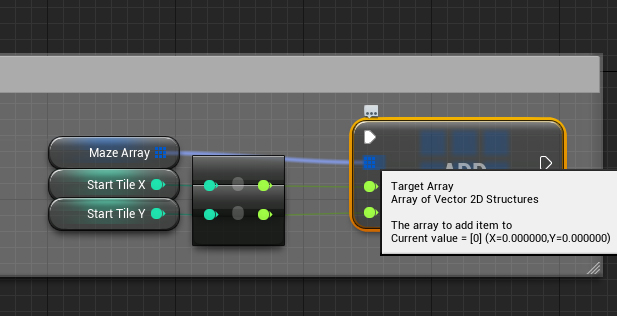Next  what we want to do it to set the CurrentTileX and Y to StartTileX and Y. Because we are going to use CurrentTile later a lot. Then we need to add a Forloop, and just for testing purpose I have just made it loop grid X multiply with the grid Y.Now the next part is where I use the Currentile. I made a function called Find next tile, and that is what is does. So Find Next Tile based on what number is in CurrentTile, then picks a random number from 0 – 3. 0 is North, 1 is East, 2 is South and 3 is West. It then uses the 5 tile check (that I talked about in part I) to see if it can go there, if it cant then it picks another random direction until it cant find one. If all 4 directions is checked as “not found”, then it sets “Stuck?” to true.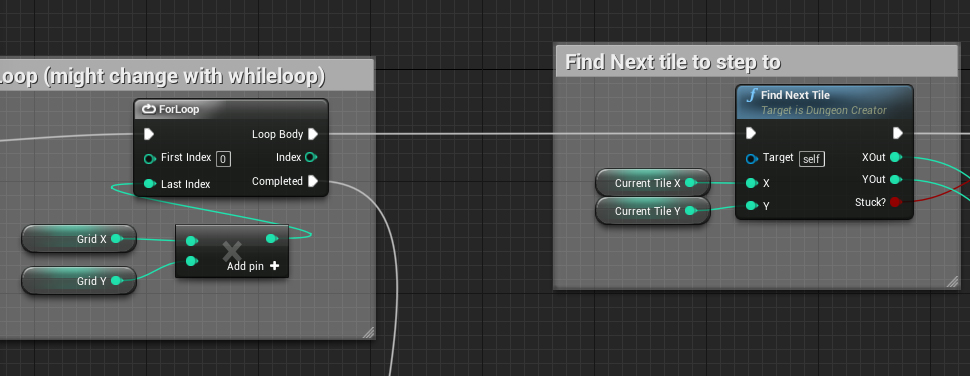If Stuck is false then it adds that tile to the Maze array. After that it sets CurrentTile to the X,Y coordinates of the new tile we just created. So next time it loops trough to try to find a tile to move to, it starts from the coordinates of the tile we just created.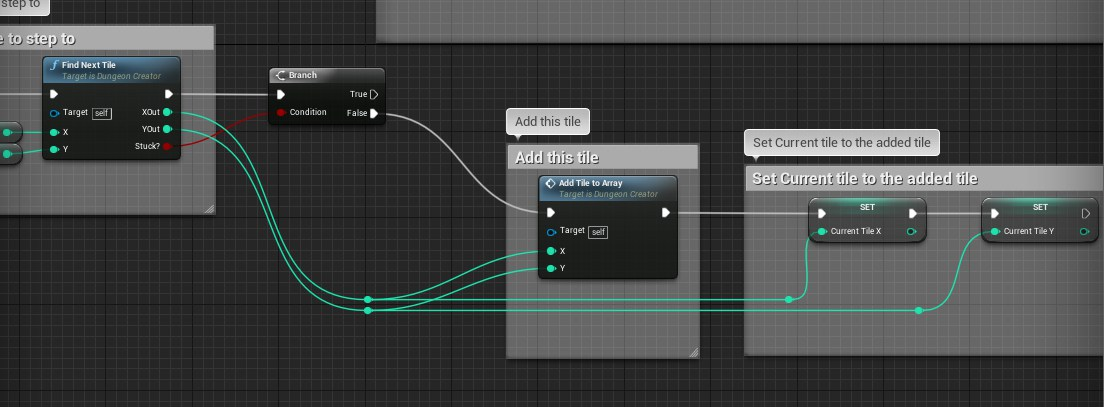Now we end up with a maze that looks like this :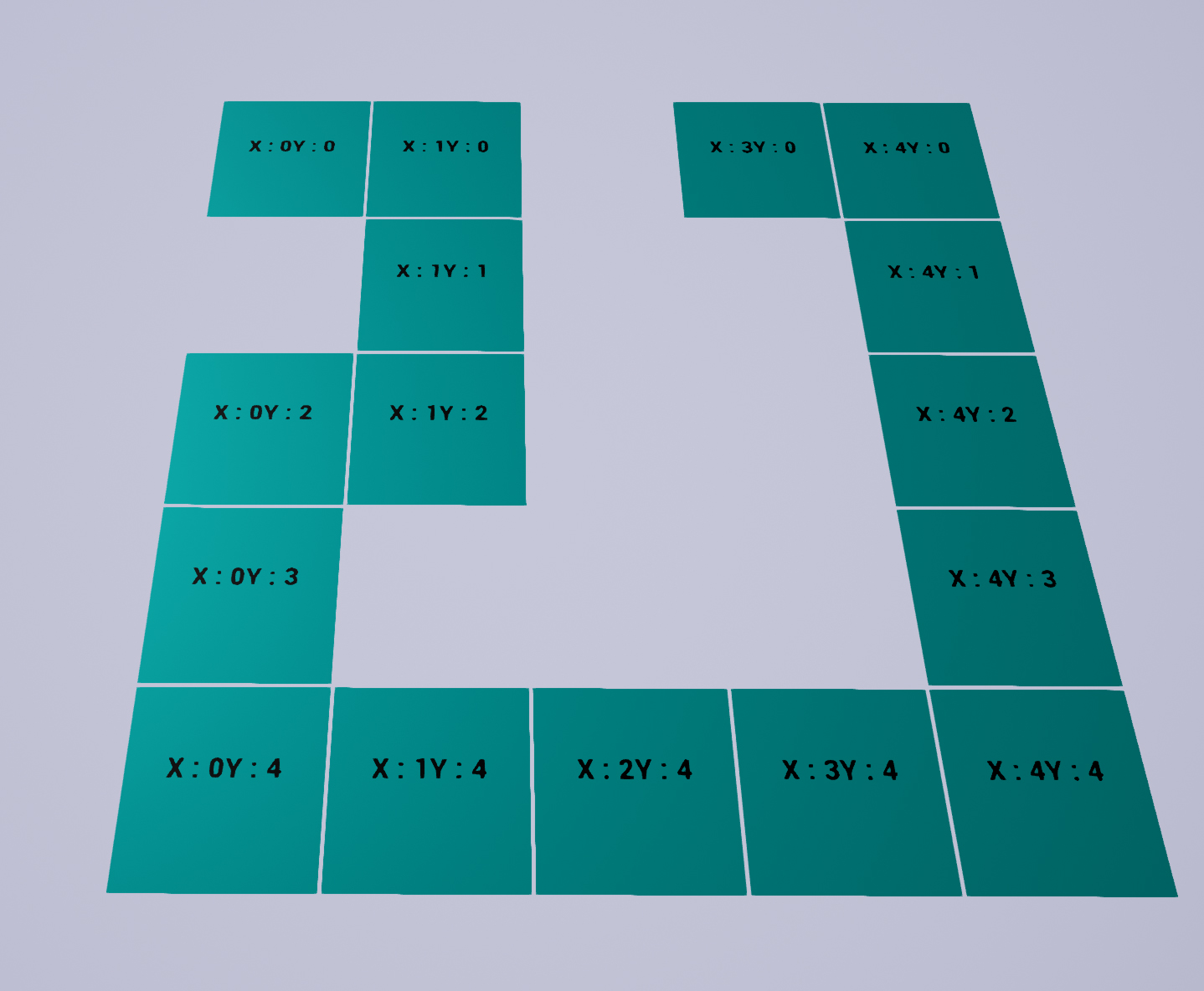Adding more complex code gives us a more interesting looking dungeon. We can make the maze expand where there is enough room. Like the screenshot below. Also you can also create a tile, between two tiles, that has one spacing to get a more connected dungeon, Second screenshot below.